# AP Calculus BC Practice Test 16

### Test Information10 questions20 minutes

Calculator Disallowed

1.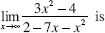2.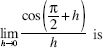3. If, for all x, f (x) = (x - 2)4 (x - 1)3, it follows that the function f has

4. Let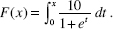Which of the following statements is (are) true?

I. F (0) = 5

II. F(2) < F(6)

III. F is concave upward.

5. If f (x) = 10x and 101.0410.96, which is closest to f (1)?

6. If f is differentiable, we can use the line tangent to f at x = a to approximate values of f near x = a. Suppose this method always underestimates the correct values. If so, then at x = a, the graph of f must be

7. The region in the first quadrant bounded by the x-axis, the y-axis, and the curve of y = e- x is rotated about the x-axis. The volume of the solid obtained is equal to

8.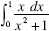is equal to

9.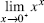10. Use the table below, which shows the values of differentiable functions f and g.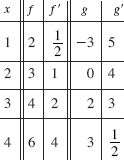If P(x) = g2 (x), then P (3) equals